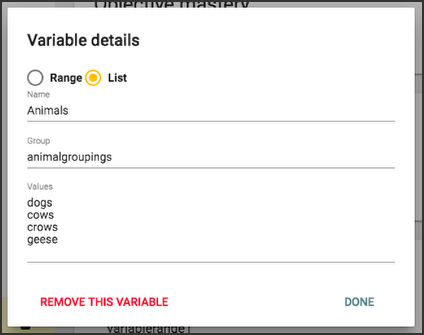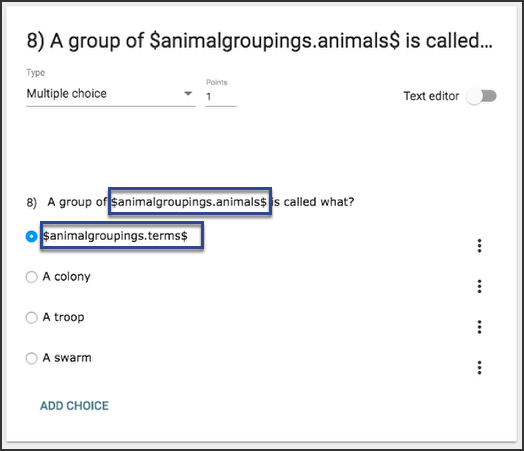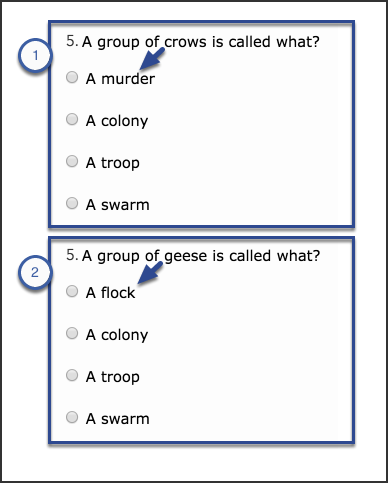# Teacher

## How do I use assessment variables?

Assessment variables allow you to create questions that use different values for each student, presenting them with varied questions and answers.

You can create two kinds of assessment variables in Buzz: ranges and lists.

## Variable ranges

Variable range values are always numbers. When you create a variable range, you provide a minimum and maximum number. When you use that variable range, Buzz randomly generates a number using those parameters.

### Create a variable range

To create a variable range:

1. Create an assessment.
2. Create an assessment question.
3. Click Add variable on the question's Variables card.1. Select Range and:
• Name the variable range (don't use spaces or special characters).
• Provide the lowest number you want included in the range in the Min field (you can use decimals and negative numbers).
• Provide the highest number you want included in the range in the Max field (you can use decimals and negative numbers).
• Provide the increments between Min and Max that you want included in the Step field. For example, if you specify 1, every whole within the parameters could be used. If you specify 2, only every other whole number can be used (you can use decimals).
2. Click Done.### Use a variable range

Once you have created a variable range, you can use it in questions and answer options.

Example

This example uses two variable ranges in a multiple choice question. Here's how they're used:

1. Place the variable range Name between dollar signs (\$) to signal Buzz to use the variable range:
``\$variablerange1\$``
1. Within a question, Buzz generates the same number for every instance of the variable range. This allows you to create answer options that use the same number that's generated in the question.
2. You can create math problems using variable range and have Buzz show the solution by:
• Typing the math problem in parentheses.
• Adding \$eval in front of the parenthesis.
• Adding a comma and a number sign (#) the end of the problem, inside the parentheses.
``\$eval(\$variablerange1\$+\$variablerange2\$,#)``
1. You can indicate how many decimal places you'd like included by adding a decimal and the desired number of places as zeros after the number sign (#) and inside the parentheses.This question is now generated differently for each assessment attempt, as in the two instances shown here.## Variable lists

Variable lists allow you to create a set of items, each of which can be swapped in at random anywhere you want in a question.

### Create a variable list

To create a variable list:

1. Create an assessment.
2. Create an assessment question.
3. Click Add variable on the question's Variables card.1. Select List and:
• Name the variable list (don't use spaces or special characters).
• Provide a Group title (don't use spaces or special characters).
• Provide the Values for the items in the list in a list.
2. Click Done.### Use a variable list

Once you have created a variable list, you can use it in questions and answer options.

Example

More than one variable list can be part of the same Group, allowing you to align items between multiple variable lists in one question. To do this, you use the same Group title for multiple lists with different Names. For example, one variable list in a Group titled animalgroupings could list animals (with Animals as the Name), and a second list with the same Group title might list the terms used for groupings of those animals (with Terms as the Name). The items align by position in the list, like this:

• Name: Animals, Group: animalgroupings, Values: dogs, cows, crows, geese
• Name: Terms, Group: animalgroupings, Values: pack, herd, murder, flock

This example uses these two variable lists (Animals and Terms) as part of the same Group (animalgroupings) in a multiple choice question. To reference the variable lists in the question, you place Group.Name between dollar signs (\$), like this:

• \$animalgroupings.animals\$
• \$animalgroupings.terms\$This question is now generated differently for each assessment attempt, as in the two instances shown here. Notice that the items from each list align correctly.